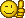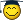# معادله درجه دومی به زبان فرترن

#### asemo0n

##### عضو جدید
سلام یه برنامه به زبان فرترن میخواستم به مشخصات زیر ممنون میشم کسی از دوستان کامپیوتر جوابمو بده .معادله درجه دومی به صورت زیر بنویسید که در ان اگر دلتای معادله بزرگتر از صفر بود مجموع ریشه های مهادله را حساب کند
مشخصات معادله:
ax^2+bx+c
a=1500-2n
b=1,n,1
c=5000\n
n=9283
دلتا=b^2-4ac

#### floe

##### کاربر بیش فعال
کاربر ممتاز
سلام
امیدوارم برنامه زیر کمکت کنه

Program main

implicit none

integer n
double precision sum

n=9283

call sumation (n, sum)

print*, sum

Pause

End

Subroutine sumation (n, sum)

implicit none

integer, intent(in) :: n
double precision, intent(out) :: sum
integer i
double precision a, b, c, delta

sum=0.

do i=1, n
a=1500.-2.*real(i)
b=real(i)
c=5000./real(i)
delta=b**2.-4.*a*c
if (delta >= 0.) then
if (i == 750) then
sum=sum-c/b
else
sum=sum-b/a
end if
else
continue
end if
end do

End

جواب میشه :

sum=5585.05928454

مرسی دوست گرامی

#### razipatala

##### عضو جدید
سلام من یه برنامه می خواستم nفاکتوریل m به توان n فاکتوریل m*n و m/n البته برای اعداد خیلی بزرگ مثلا 30 40 رقمی و بالاتر اگه اینا رو نبرم تو برنامه نویسی می افتم اگه کمکم کنید ممنون میشم.

#### samira19

##### عضو جدید
سلام
شما درباره ساختار درخت پارسه توی زبان فرترن چیزی میدونین؟
یه مثال واسه درخت پارسه میخوام ولی پیدا نمیکنم.

##### عضو جدید
سلام
من به سابروتین حل سیستم های معادلات خطی به روش گاوس-سایدل احتیاج دارم
کسی می تونه به من کمک کنه؟

#### don_pedram

##### عضو جدید
PROGRAM Equation_Set_Solution_by_Gauss_Sidel_Method

!This program have been written by Pedram Niakan

IMPLICIT NONE

DOUBLE PRECISION :: er,sum

DOUBLE PRECISION,ALLOCATABLE :: a,,b),x),y)
INTEGER :: i,j,k,n

!Declaration of the matrix of factors

WRITE (*,*) "Please enter number of Equations & Variables:"

ALLOCATE (a(1:n,1:n))
ALLOCATE (b(1:n))
ALLOCATE (x(1:n),y(1:n))

WRITE (*,*) "Please generat the matrix of factors:"

DO i=1,n
DO j=1,n
WRITE (*,*) "Please enter array (",i,",",j,") for matrix of factors"
END DO
WRITE (*,*) "Please enter array (",i,") for matrix of values"
END DO

WRITE (*,*) "The 3 required matrixes for these equations system have been generated successfully"
WRITE (*,*)""

WRITE (*,*) "Please enter",n," probable numbers:"
DO i=1,n,1
WRITE (*,*) "Please enter the probable root: x(",i,")="
x(i)=y(i)
END DO

k=1

10 DO i=1,n,1
sum=b(i)/a(i,i)
DO j=1,n,1
IF (j/=i) THEN
x(i)=(-a(i,j)*x(j))/a(i,i)+sum
sum=x(i)
END IF
END DO
END DO

er=0
DO i=1,n,1
er=DABS((x(i)-y(i))/y(i))+er
END DO

IF (er<=0.010000000) THEN !Accuracy
DO i=1,n,1
WRITE (*,*) "Accurate Root: X(",i,")=",x(i)
END DO
WRITE (*,*) "Number of retries:",k
ELSE
DO i=1,n,1
WRITE (*,*) "Approximate Root: X(",i,")=",x(i)
y(i)=x(i)
END DO
WRITE (*,*) "Try:",k
k=k+1
PAUSE
GO TO 10
END IF

PAUSE

END PROGRAM

من برنامش رو نوشتم لطفا خودت به سابروتین تبدیلش کن

#### artarya

##### عضو جدید
سلام
چطور میتونیم تو برنامه فرترن یه فایل دیتا رو به رشته تبدیل کنیم؟

#### Tarikh-S

##### عضو جدید
لطفا راهنمايي كنيد.
1- برنامه اي كه يك ماتريس n*n را در نظر گرفته و آنرا به توان 16 برساند
2- برنامه اي كه دترمينان يك ماتريس مربع n*n را حساب كند.

#### yazdipanah

##### عضو جدید
سلام برنامه ای در زبان فرترن می خواستم که کاربر دو نقطه A(x1,y1) و B(x2,y2) را وارد کند و برنامه معادله خط را به کاربر بدهد.لطفا کمکم کنید!
شیب خط: m=(y2-y1)/(x2-x1)

معادله خط= y-y1=m(x-x1)

#### ami92

##### عضو جدید
سلام من دستور حل معادله درجه 3 با برنامه فرترن رو میخام .لطفا کمکم کنین .ممنون

#### mehrz67

##### عضو جدید
با سلام
برای حل معادلتون باید همه جملات رو ببرین یه طرف بعد ریشه اونو با رروشها ی نصف کردن و نیوتون رافسون و .... بدست بیاری
معادلتو بگو شاید بتونم کمک کنم